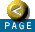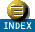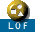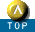GEATbx: Main page  Tutorial  Algorithms  M-functions  Parameter/Options  Example functions  www.geatbx.com

# Evolutionary Algorithms 1 Introduction# 1 Introduction

Fig. 1-1: Problem solution using evolutionary algorithmsDifferent main schools of evolutionary algorithms have evolved during the last 40 years: genetic algorithms, mainly developed in the USA by J. H. Holland [Hol75], evolutionary strategies, developed in Germany by I. Rechenberg [Rec73] and H.-P. Schwefel [Sch81] and evolutionary programming [FOW66]. Each of these constitutes a different approach, however, they are inspired by the same principles of natural evolution. A good introductory survey can be found in [Fdb94a].

This document describes algorithms of evolutionary algorithms. In Chapter 2 a short overview of the structure and basic algorithms of evolutionary algorithms is given. Chapter 3 describes selection. In Chapter 4 the different recombination algorithms are presented. Chapter 5 explains mutation and Chapter 6 reinsertion.

Chapter 8 covers parallel implementations of evolutionary algorithms especially the regional population model employing migration in detail. The application of multiple/different strategies during an optimization including competition between subpopulations is discussed in Chapter 9.

Chapter 10 explains how complete optimization algorithms can be created from the different evolutionary operators. The respective options are discussed in detail. Each of the presented optimization algorithms represents an evolutionary algorithm.

Chapter 11 lists all the used references and a large number of other publications from the field of Evolutionary Algorithms.GEATbx: Main page  Tutorial  Algorithms  M-functions  Parameter/Options  Example functions  www.geatbx.com

This document is part of version 3.8 of the GEATbx: Genetic and Evolutionary Algorithm Toolbox for use with Matlab - www.geatbx.com.
The Genetic and Evolutionary Algorithm Toolbox is not public domain.